Geometry

# Geometry Warmups: Level 2 Challenges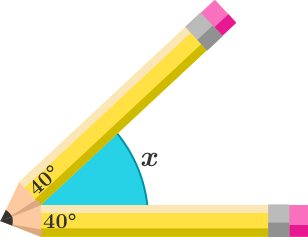Hazri aligned two identical pencils in such a way that the pencil tips are stuck together as shown in the 2D (flat) diagram above.

If the angles formed by the pencil tips in the 2D diagram are both $40 ^ \circ$, then what is the value of $x$ in degrees?

(Assume the pencils have a rectangular body and have their tips resembling isosceles triangles)

What is the area of the large square?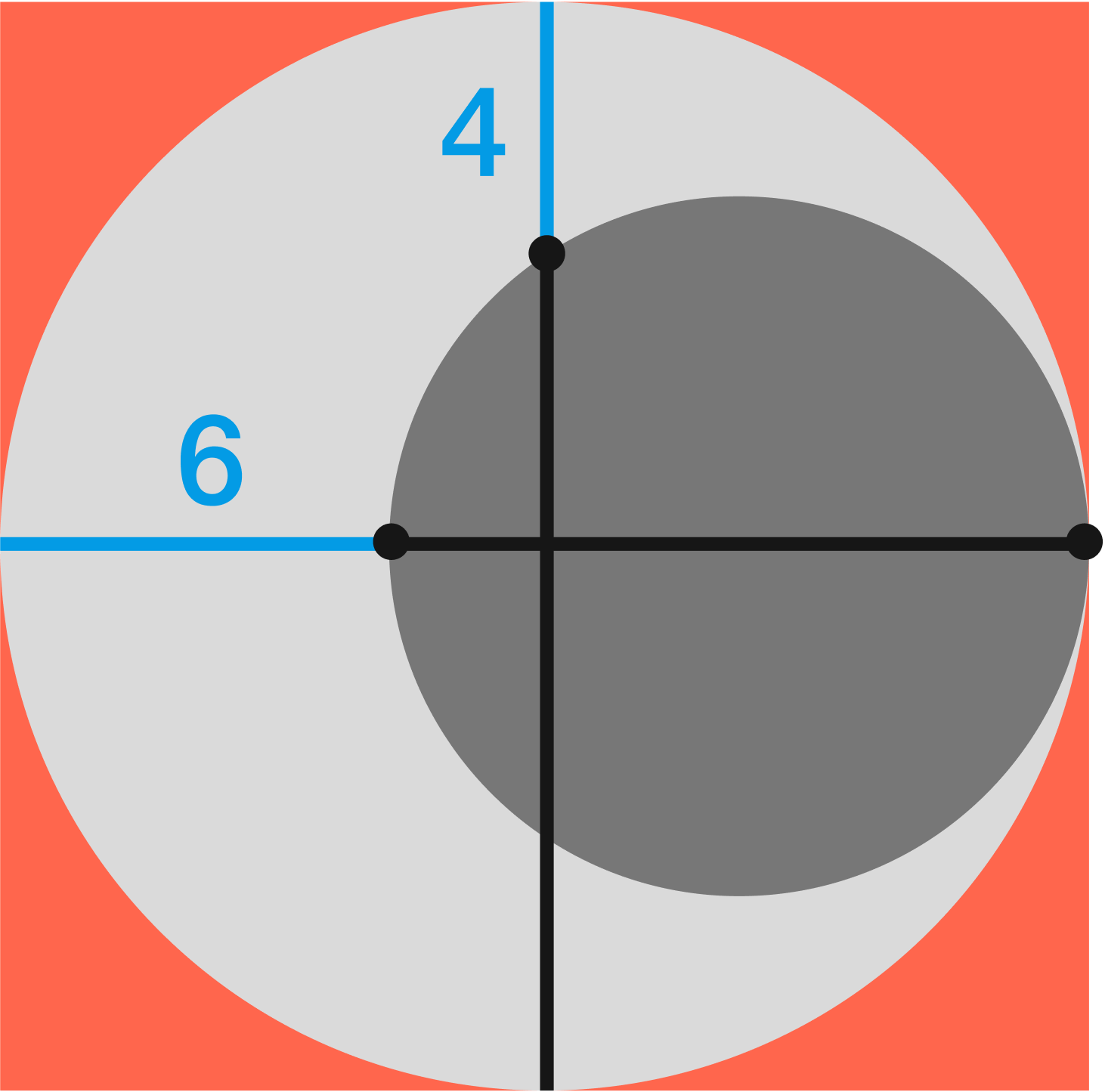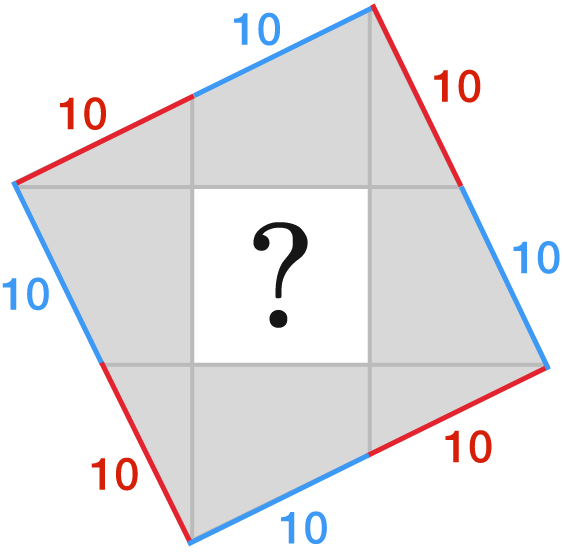Line segments are drawn from the vertices of the large square to the midpoints of the opposite sides to form a smaller, white square.

If each red or blue line-segment measures $10$ m long, what is the area of the smaller, white square in m$^{2}?$

Which route (on Earth's surface) has a shorter distance?

Route A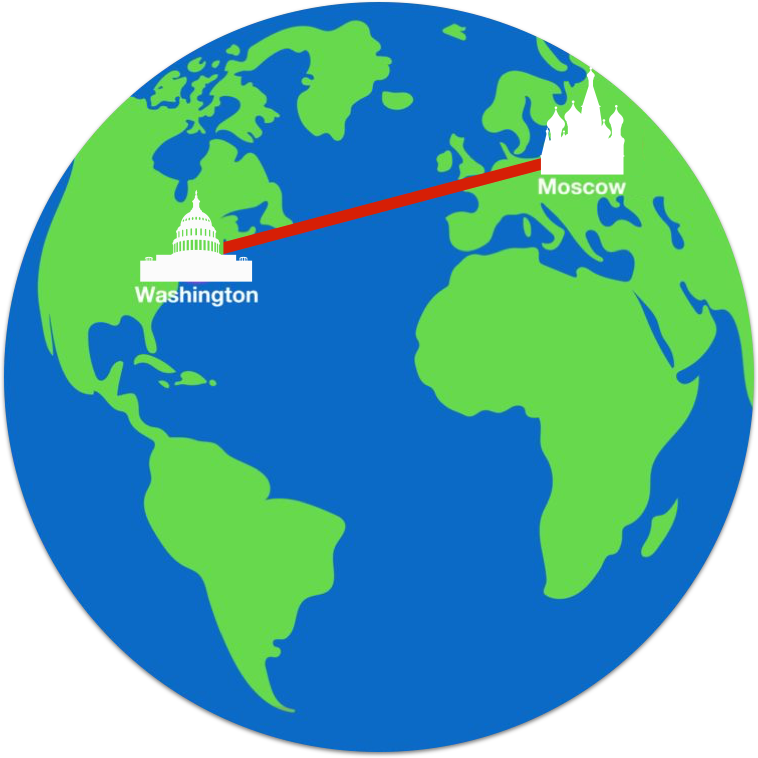Route B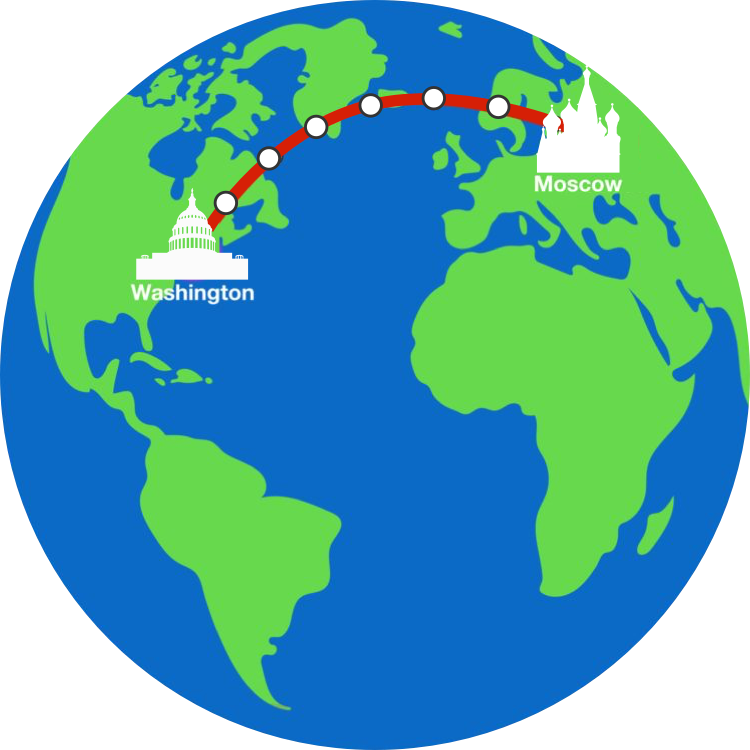Note: The images are a 2-D projection of the route on a globe, which is why the continents are in a slightly different shape. In particular, Greenland isn't as huge as compared to its representation using the cylindrical map projection.

A vertical 32-meter bamboo stick was broken by the wind, such that the tip meets the ground at a point 16 meters from the base. At what height above the ground (in meters) was it broken?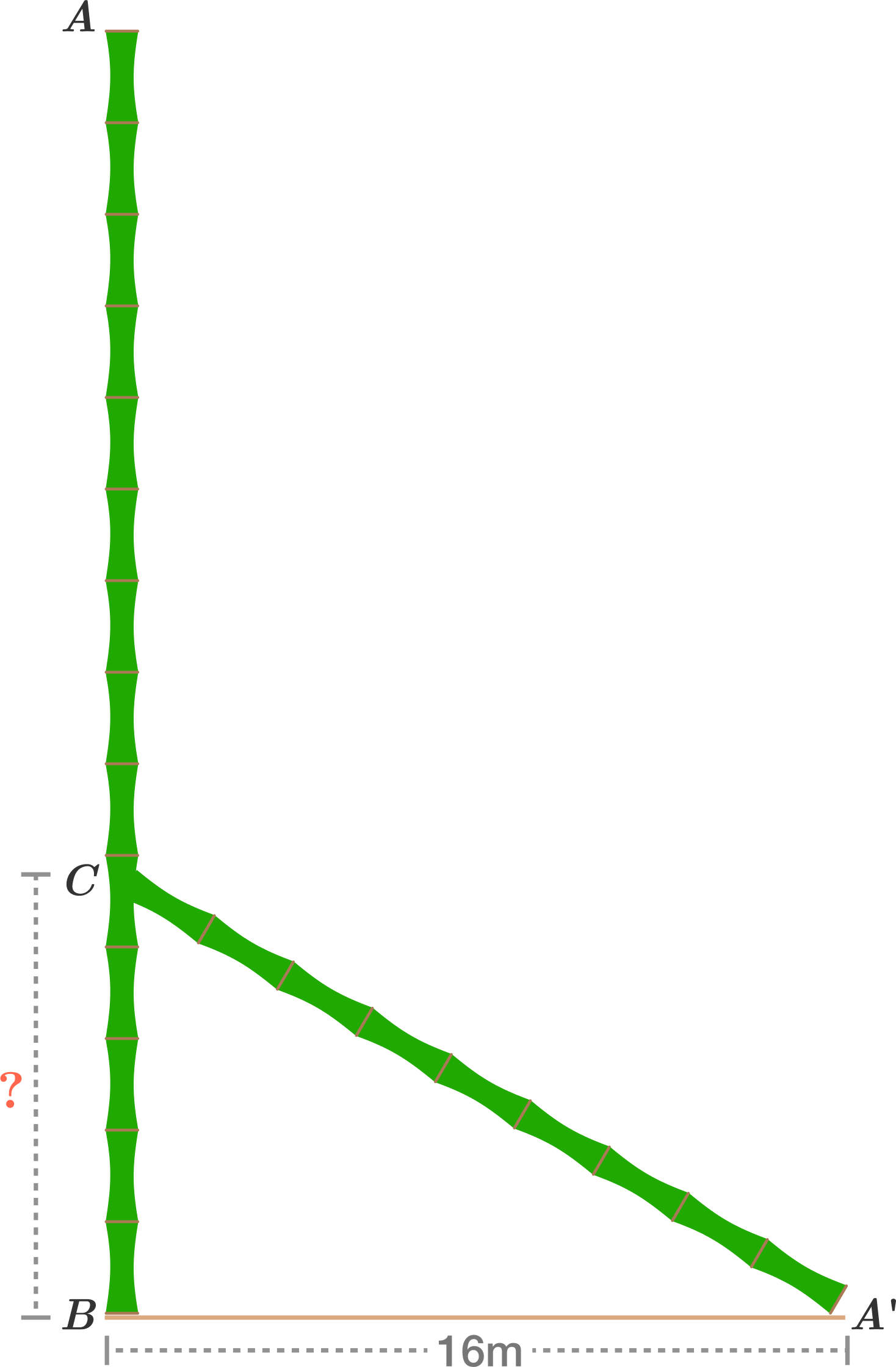×